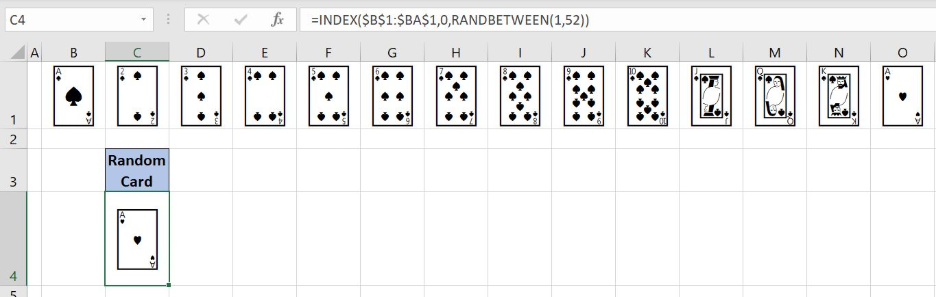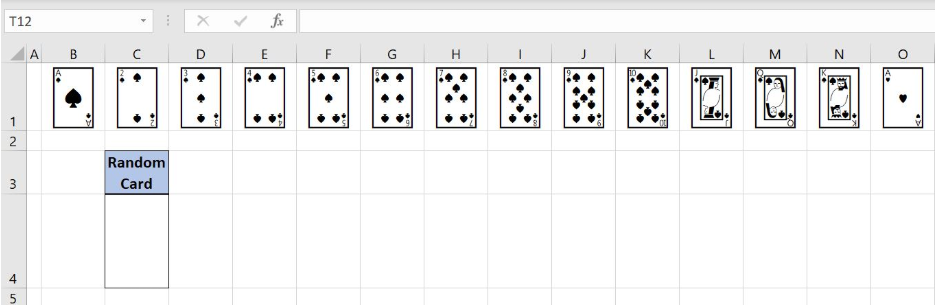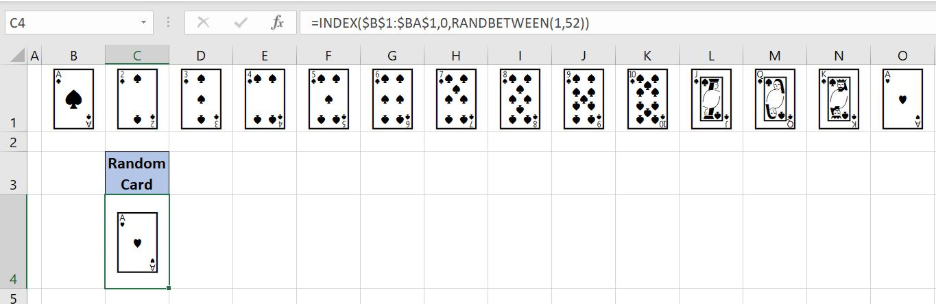Get instant live expert help with Excel or Google Sheets“My Excelchat expert helped me in less than 20 minutes, saving me what would have been 5 hours of work!”

#### Post your problem and you’ll get expert help in seconds.

Your message must be at least 40 characters
Our professional experts are available now. Your privacy is guaranteed.

# How to Create a Random Playing Card Generator in Excel

Excel allows us to get a random card using the INDEX and RANDBETWEEN functions. This step by step tutorial will assist all levels of Excel users to create a random playing card generator in Excel.Figure 1. The final result of the random playing card generator

## Syntax of the INDEX formula

The generic formula for the INDEX function is:

`=INDEX(array, row_num, column_num)`

The parameters of the INDEX function are:

• array – a range of cells where we want to get a data
• row_num – a number of a row in the array for which we want to get a value
• column_num – a column in the array which returns a value.

## Syntax of the RANDBETWEEN formula

`=RANDBETWEEN(bottom, top)`

The parameters of the RANDBETWEEN function are:

• bottom – a value from which we want to get a random value
• top – a value to which we want to get a random value

## Setting up Our Data for the Random Playing Card Generator

In the array B1:BA1 we have 52 playing cards while in the cell C4 will be placed the random card result.

To insert the card in the cell, we need to follow these steps:

• Select cell B1 and click on it
• Under the tab Insert and section Symbols choose Symbol
• Click Symbols and choose Font Segoe UI Symbol
• Find the card, click Insert tab and then Close tab
• Repeat this steps for other 51 cards

Figure 2. Data that we will use in the example

## Get a Random Playing Card Generator in Excel

In the cell C4, we want to get the random playing card from the range B1:BA1.

The formula looks like:

`=INDEX(\$B\$1:\$BA\$1,0,RANDBETWEEN(1,52))`

In the INDEX function, the parameter array is the cell range \$B\$1:\$BA\$1. The row_num is 0 while the column_num is the formula `RANDBETWEEN(1,52)`. The RANDBETWEEN parameter bottom is 1, while the top is 52.

To apply the formula, we need to follow these steps:

• Select cell C4 and click on it
• Insert the formula: `=INDEX(\$B\$1:\$BA\$1,0,RANDBETWEEN(1,52))`
• Press enter.

Figure 3. Get a random playing card with INDEX and RANDBETWEEN functions

With INDEX function we want to get the card from the array B1:BA1. The playing card in the cell C3 should be the random card. That’s why we use the RANDBETWEEN function in the column argument of the INDEX function. The RANDBETWEEN function result is 14 and final formula result is the card from the cell O1.

Most of the time, the problem you will need to solve will be more complex than a simple application of a formula or function. If you want to save hours of research and frustration, try our live Excelchat service! Our Excel Experts are available 24/7 to answer any Excel question you may have. We guarantee a connection within 30 seconds and a customized solution within 20 minutes.

Solution examplesI need help on index and match where the return is not correct
Solved by B. B. in 20 minsI have a database extract that is showing the same clients multiple times in the list. How can I write a formula in excel to look at the column with the client names and return only unique records on another sheet? I want to run this over several files so wanting to avoid using pivot tables which is how I would normal do this.
Solved by X. J. in 20 minsHi, I have created a drop down list and in another cell I have used an IF function which is dependent on the drop down list. However, when i change the selected option from the drop down list, the IF function cell does not update accordingly
Solved by C. C. in 17 minsI work shift work as do about 100 other guys. All I want to do is type in the days date (Or just open the sheet and it will have the days date) and it has on there who is working that day/night. Pretty simple I would think...And if I change the date to, let's say, a month down the road, it pulls in the guys working that day... That all...Thx
Solved by O. E. in 20 minsindex and match with duplicates. I need to use another column as a reference, so my return value has two match the value of two things for it to return
Solved by E. H. in 60 mins